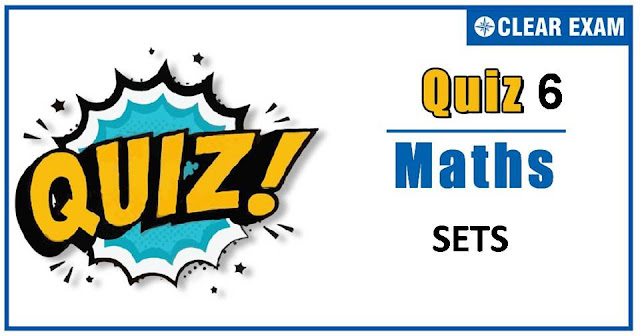## [LATEST]\$type=sticky\$show=home\$rm=0\$va=0\$count=4\$va=0

As per analysis for previous years, it has been observed that students preparing for JEE MAINS find Mathematics out of all the sections to be complex to handle and the majority of them are not able to comprehend the reason behind it. This problem arises especially because these aspirants appearing for the examination are more inclined to have a keen interest in Mathematics due to their ENGINEERING background.

Furthermore, sections such as Mathematics are dominantly based on theories, laws, numerical in comparison to a section of Engineering which is more of fact-based, Physics, and includes substantial explanations. By using the table given below, you easily and directly access to the topics and respective links of MCQs. Moreover, to make learning smooth and efficient, all the questions come with their supportive solutions to make utilization of time even more productive. Students will be covered for all their studies as the topics are available from basics to even the most advanced.

Q1. Let R and S be two equivalence relations on a set A. Then,
•  R∪S is an equivalence relation on A
•  R∩S is an equivalence relation on A
•  R-S is an equivalence relation on A
•  None of these
Solution
No solution available

Q3. In a certain town 25% families own a cell phone, 15% families own a scooter and 65%
families own neither a cell phone nor a scooter. If 1500 families own both a cell phone and
a scooter, then the total number of families in the town is
•  10000
•  20000
•  30000
•  40000

•  2
•  3
•  4
•  6

Q5.If A={ϕ,{ϕ} }, then the power set of A is
•   A
•  {ϕ,{ϕ},A}
•  {ϕ,{ϕ},{{ϕ} },A}
•  None of these
Solution
No solution available

Q6. For any two sets A and B, if A∩X=B∩X=ϕ and A∪X=B∪X for some set X, then
•  A-B=A∩B
•  A=B
•  B-A=A∩B
•  None of these
Solution
Given, A∩X=B∩X=ϕ ⇒A and X,B and X are disjoint sets. Also, A∪X=B∪X⇒A=B

Q7.Let L be the set of all straight lines in the Euclidean plane. Two lines l1 and l2 are said to be related by the relation R iff l1 is parallel to l2. Then, the relation R is not
• Reflexive
•  Symmetric
•  Transitive
•  None of these
Solution
No solution available

Q9.Let R be a relation on the set N of natural numbers defined by nRm⇔n is a factor of m(i.e. n | m). Then, R is
•  Reflexive and symmetric
•  Transitive and symmetric
•  Equivalence
•  Reflexive, transitive but not symmetric
Solution
Since n|n for all n∈N. Therefore, R is reflexive. Since 2|6 but 6∤2, therefore R is not symmetric Let n R m and m R p ⇒n R m and m R p ⇒n|m and m|p⇒n|p⇒n R p So, R is transitive

Q10. If A and B are two sets such that n(A)=7,n(B)=6 and (A∩B)≠ϕ. The least possible value of n(A Δ B), is
•  11
•  7
•  6
•  13
Solution
We have, A ∆ B=(A∪B)-(A∪B) ⇒n(A ∆ B)=n(A)+n(B)-2 n(A∩B) So, n(A ∆ B) is greatest when n(A∩B) is least It is given that A∩B≠ϕ. So, least number of elements in A ∩B can be one ∴ Greatest possible value of n(A ∆ B) is 7+6-2×1=11#### Written by: AUTHORNAME

AUTHORDESCRIPTION## Want to know more

Please fill in the details below:

## Latest NEET Articles\$type=three\$c=3\$author=hide\$comment=hide\$rm=hide\$date=hide\$snippet=hide

Name

ltr
item
BEST NEET COACHING CENTER | BEST IIT JEE COACHING INSTITUTE | BEST NEET, IIT JEE COACHING INSTITUTE: SETS-QUIZ-6
SETS-QUIZ-6
https://1.bp.blogspot.com/-57RnRm355mg/X5QqutKFRTI/AAAAAAAAFOQ/XBeP2RtE_IElIDQndER_N8ocnm5U7nSIQCLcBGAsYHQ/w640-h336/quiz.jpg
https://1.bp.blogspot.com/-57RnRm355mg/X5QqutKFRTI/AAAAAAAAFOQ/XBeP2RtE_IElIDQndER_N8ocnm5U7nSIQCLcBGAsYHQ/s72-w640-c-h336/quiz.jpg
BEST NEET COACHING CENTER | BEST IIT JEE COACHING INSTITUTE | BEST NEET, IIT JEE COACHING INSTITUTE
https://www.cleariitmedical.com/2020/10/SETS-QUIZ-6.html
https://www.cleariitmedical.com/
https://www.cleariitmedical.com/
https://www.cleariitmedical.com/2020/10/SETS-QUIZ-6.html
true
7783647550433378923
UTF-8